# Bubble chart in ggplot2

A bubble chart is a type of scatter plot where the points are scaled based on a variable.

## Sample data

In order to create a bubble plot in ggplot2 you will need a data frame containing the coordinates of the points (`x` and `y`) and a variable to map the sizes of the bubbles (`size`). In addition, you can have a variable representing groups or labels.

``````# Sample data
x = c(2, 5, 7, 3, 6, 1, 9, 2)
y = c(2.2, 2, 1, 2, 1, 4, 1, 6)
size = c(100, 30, 50, 250, 120, 140, 80, 36)
group = c("A", "A", "A", "B", "C", "B", "D", "B")

# Data frame
df <- data.frame(x = x, y = y, size = size, group = group)``````

## Bubble plots in ggplot2 with `geom_point` and `scale_size` or `scale_size_area`

In order to create a basic bubble chart in ggplot2 you will need to pass the coordinates and the variable that represents sizes to the `aes` function and then use the `geom_point` function.

``````# install.packages("ggplot2")
library(ggplot2)

ggplot(df, aes(x = x, y = y, size = size)) +
geom_point()``````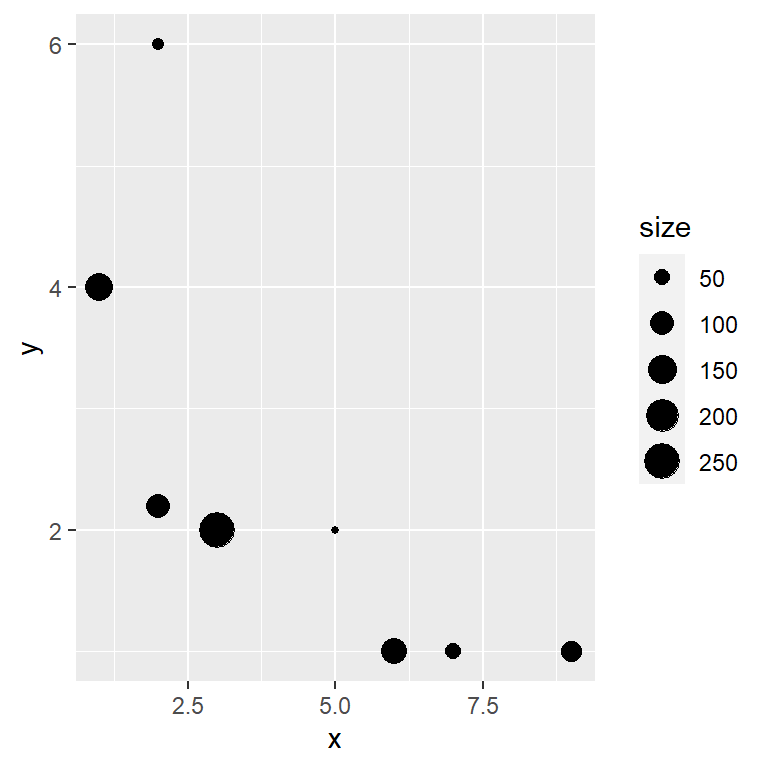Resizing the bubbles

However, the previous plot can be improved making use of the `scale_size` function, as the default bubbles are small. This function scales the size of the bubbles to a specific range of sizes. Note that you can provide a name that will be shown in the legend of the chart.

``````# install.packages("ggplot2")
library(ggplot2)

ggplot(df, aes(x = x, y = y, size = size)) +
geom_point() +
scale_size(name = "Size", range = c(3, 15))``````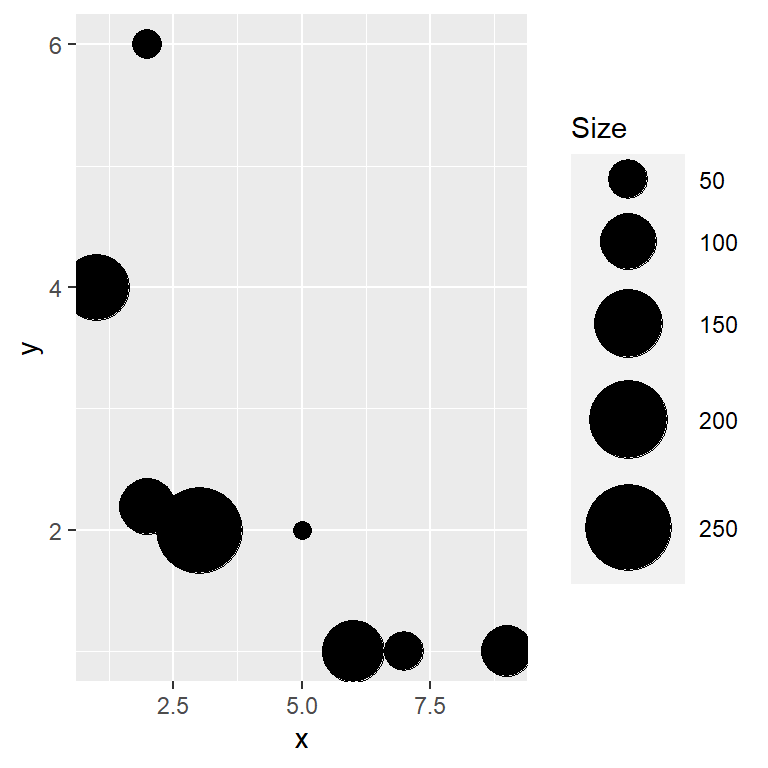scale_size_area

An alternative function to create bubble graphs is `scale_size_area` that ensures that a value of zero is mapped to a size of 0 and instead of a range a maximum size must be specified with `max_size`. This is more useful when the size represents a count of observations.

``````# install.packages("ggplot2")
library(ggplot2)

ggplot(df, aes(x = x, y = y, size = size)) +
geom_point() +
scale_size_area(max_size = 10)``````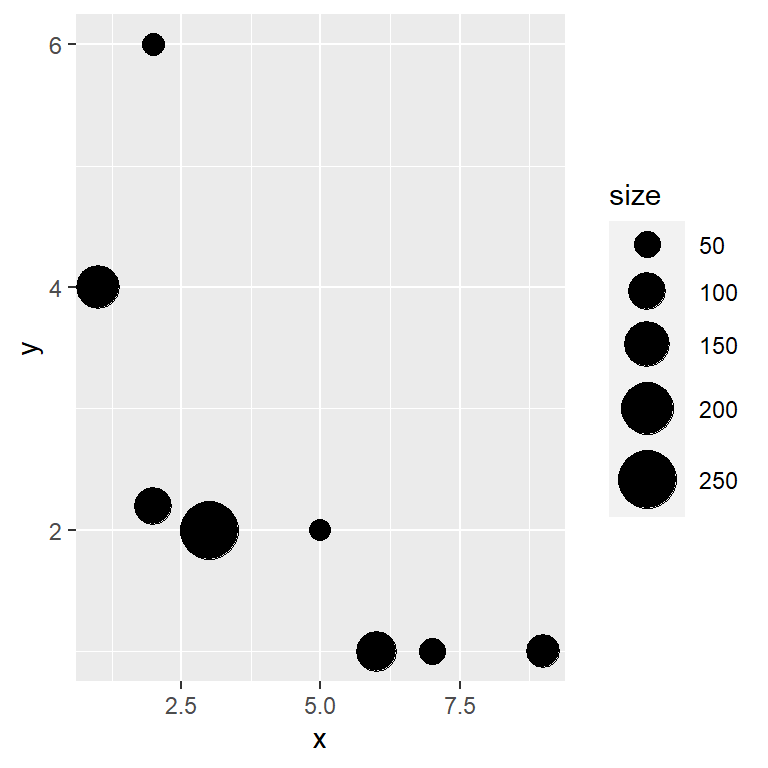Labelling each bubble

With the `geom_text` or `geom_label` functions you can add texts to the bubbles. Recall that the functions provide the `nudge_x` and `nudge_y` arguments to customize the position of the texts or labels.

``````# install.packages("ggplot2")
library(ggplot2)

ggplot(df, aes(x = x, y = y, size = size)) +
geom_point() +
geom_text(label = group, nudge_x = 0.5, color = "red") +
scale_size(name = "Size", range = c(3, 15))``````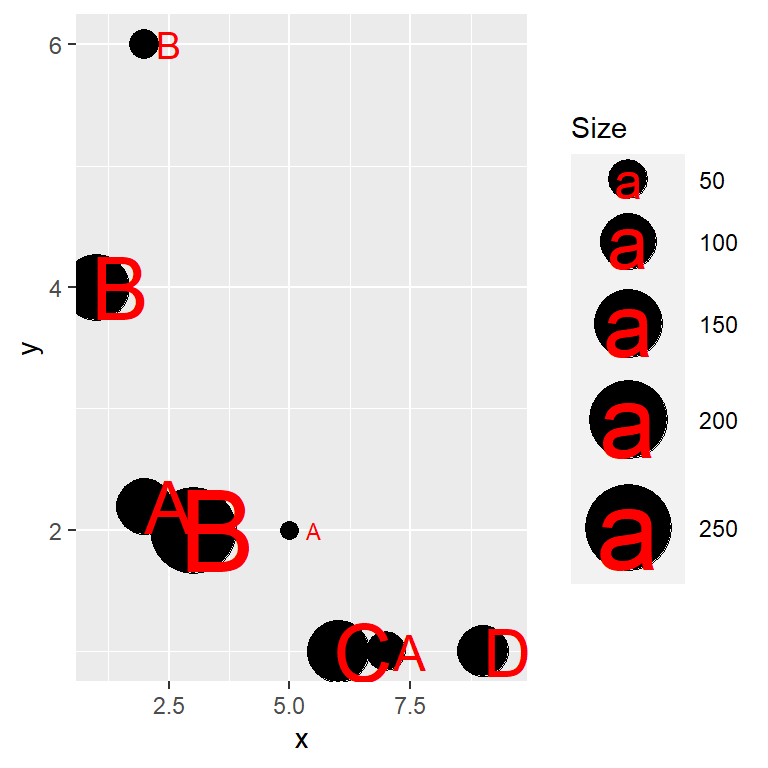Turn off the legend or change its position

It is possible to remove the legend or change its position with the `legend.position` argument of the `theme` function.

``````# install.packages("ggplot2")
library(ggplot2)

ggplot(df, aes(x = x, y = y, size = size)) +
geom_point() +
scale_size(name = "Size", range = c(3, 15)) +
theme(legend.position = "none")``````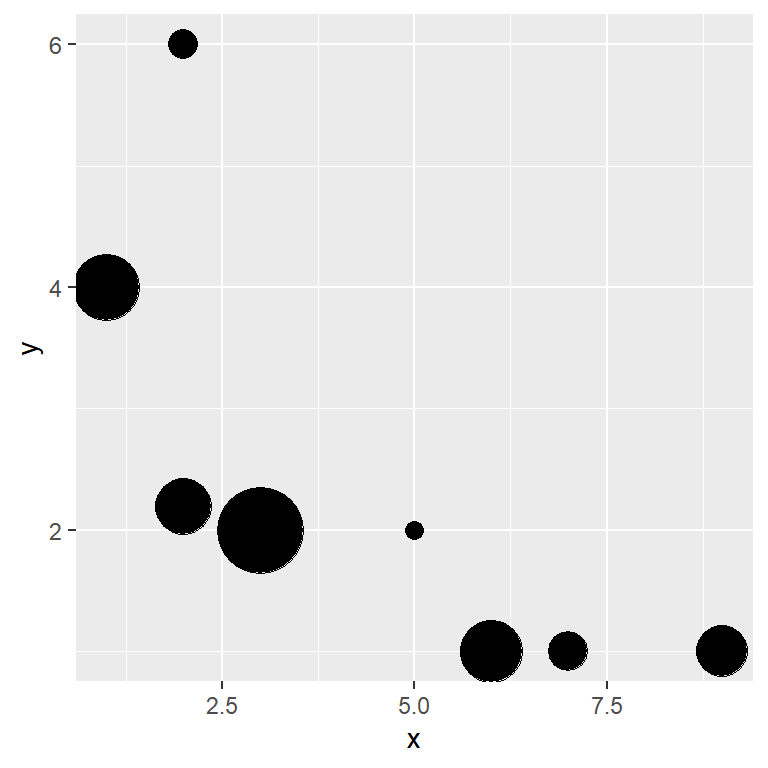## Bubble colors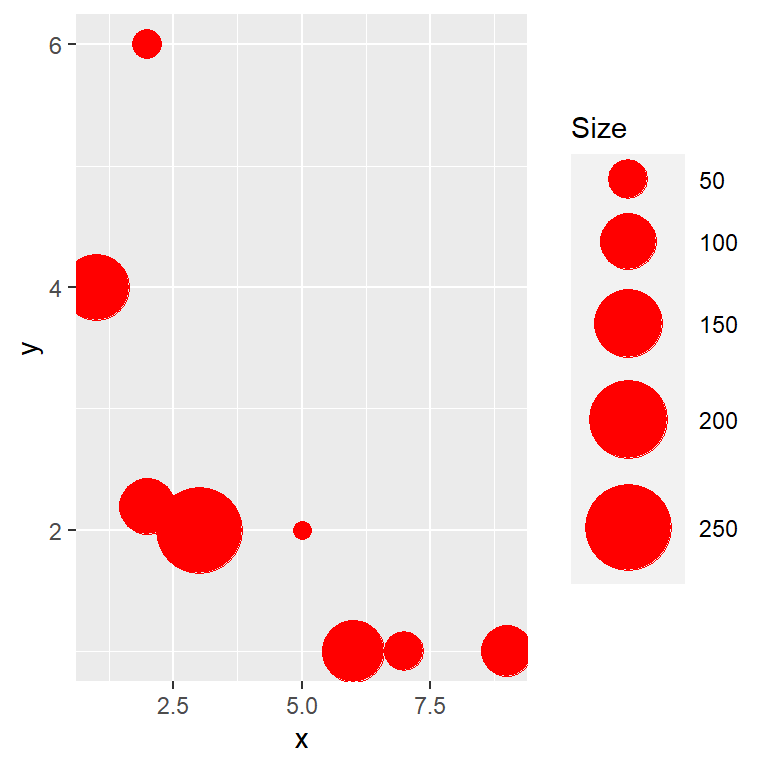The default black color of the bubbles can be customized through the `color` argument of the `geom_point` function, specifying a new color.

``````# install.packages("ggplot2")
library(ggplot2)

ggplot(df, aes(x = x, y = y, size = size)) +
geom_point(color = "red") +
scale_size(name = "Size", range = c(3, 15))``````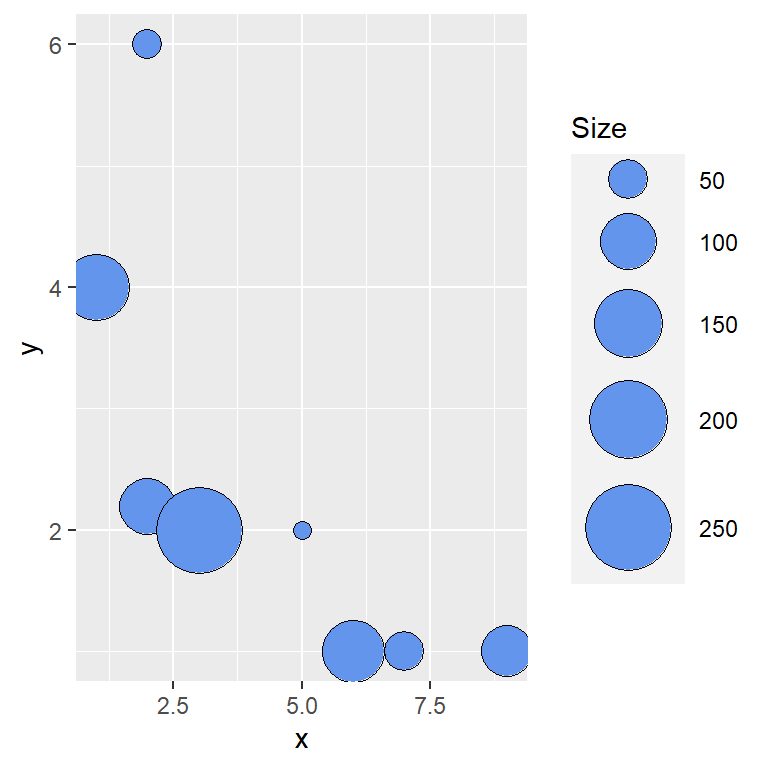Fill and border color of the bubbles

Note that there are some symbols that can handle both a fill color and a border color, such as the symbol 21.

``````# install.packages("ggplot2")
library(ggplot2)

ggplot(df, aes(x = x, y = y, size = size)) +
geom_point(fill = "cornflowerblue", color = "black", shape = 21) +
scale_size(name = "Size", range = c(3, 15))``````Color transparency

You can also control the level of transparency of the colors with `alpha`, which ranges from 0 (transparent) to 1 (opaque).

``````# install.packages("ggplot2")
library(ggplot2)

ggplot(df, aes(x = x, y = y, size = size)) +
geom_point(color = "red", alpha = 0.5) +
scale_size(name = "Size", range = c(3, 15))``````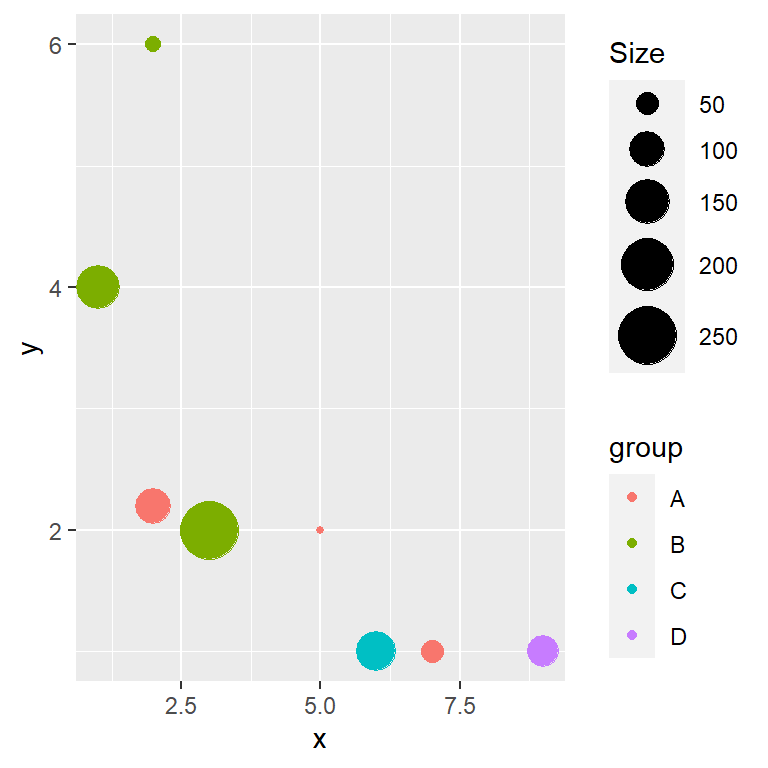Color based on a categorical variable

If you have a categorical variable that represents groups you can pass the name of that variable to `color` inside `aes`, so each group of bubbles will be filled with a different color. Use `scale_color_manual` or an equivalent function if you want to customize the default colors.

``````# install.packages("ggplot2")
library(ggplot2)

ggplot(df, aes(x = x, y = y, size = size, color = group)) +
geom_point() +
scale_size(name = "Size", range = c(1, 10))``````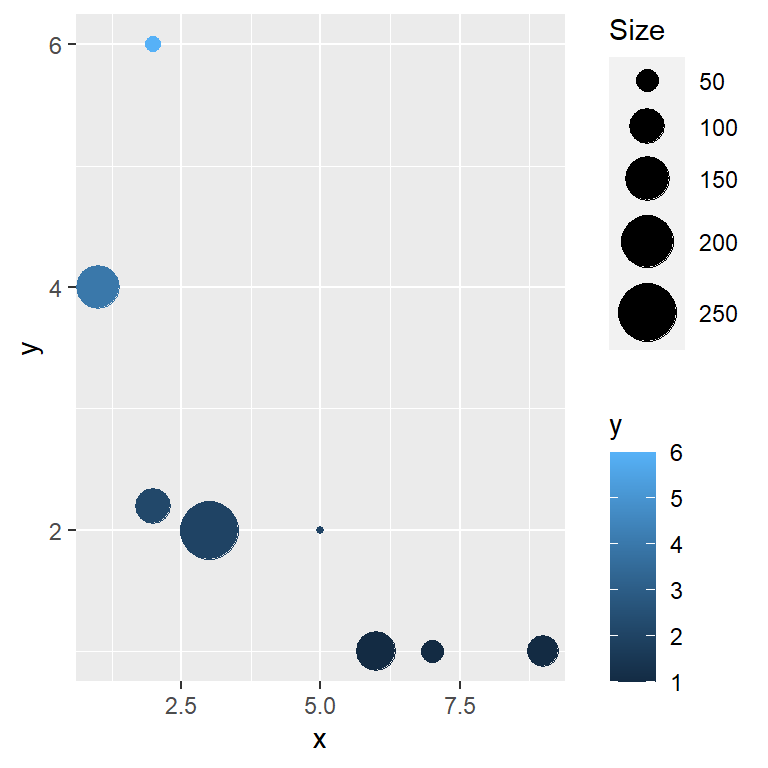Color based on a continuous variable

The color of the bubbles can also be mapped to a continuous variable. In the following example we are using the `y` variable and hence a gradient color will be applied based on that variable. Use `scale_color_gradient`, `scale_color_gradient2` or `scale_color_gradientn` to customize the color palette.

``````# install.packages("ggplot2")
library(ggplot2)

ggplot(df, aes(x = x, y = y, size = size, color = y)) +
geom_point() +
scale_size(name = "Size", range = c(1, 10))``````